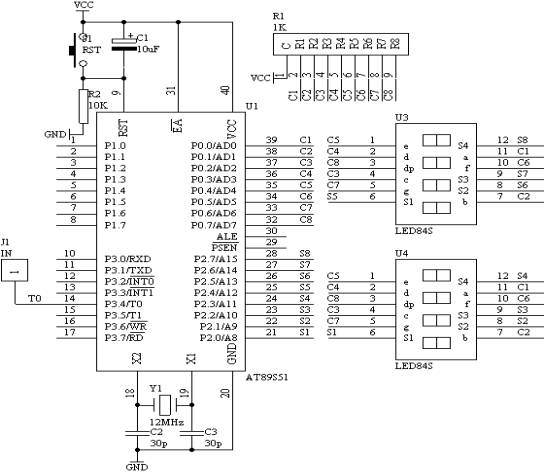进入框架页面

31． 6位数显频率计数器

1． 实验任务

2． 电路原理图3． 系统板上硬件连线

（1）． 把“单片机系统”区域中的P0.0－P0.7与“动态数码显示”区域中的ABCDEFGH端口用8芯排线连接。

（2）． 把“单片机系统”区域中的P2.0－P2.7与“动态数码显示”区域中的S1S2S3S4S5S6S7S8端口用8芯排线连接。

（3）． 把“单片机系统”区域中的P3.4（T0）端子用导线连接到“频率产生器”区域中的WAVE端子上。

4． 程序设计内容

（1）． 定时/计数器T0和T1的工作方式设置，由图可知，T0是工作在计数状态下，对输入的频率信号进行计数，但对工作在计数状态下的T0，最大计数值为fOSC/24，由于fOSC＝12MHz，因此：T0的最大计数频率为250KHz。对于频率的概念就是在一秒只数脉冲的个数，即为频率值。所以T1工作在定时状态下，每定时1秒中到，就停止T0的计数，而从T0的计数单元中读取计数的数值，然后进行数据处理。送到数码管显示出来。

（2）． T1工作在定时状态下，最大定时时间为65ms，达不到1秒的定时，所以采用定时50ms，共定时20次，即可完成1秒的定时功能。

5． C语言源程序
#include <AT89X52.H>
unsigned char code dispbit[]={0xfe,0xfd,0xfb,0xf7,0xef,0xdf,0xbf,0x7f};
unsigned char code dispcode[]={0x3f,0x06,0x5b,0x4f,0x66,
0x6d,0x7d,0x07,0x7f,0x6f,0x00,0x40};
unsigned char dispbuf={0,0,0,0,0,0,10,10};
unsigned char temp;
unsigned char dispcount;
unsigned char T0count;
unsigned char timecount;
bit flag;
unsigned long x;

void main(void)
{
unsigned char i;

TMOD=0x15;
TH0=0;
TL0=0;
TH1=(65536-4000)/256;
TL1=(65536-4000)%256;
TR1=1;
TR0=1;
ET0=1;
ET1=1;
EA=1;

while(1)
{
if(flag==1)
{
flag=0;
x=T0count*65536+TH0*256+TL0;
for(i=0;i<8;i++)
{
temp[i]=0;
}
i=0;
while(x/10)
{
temp[i]=x%10;
x=x/10;
i++;
}
temp[i]=x;
for(i=0;i<6;i++)
{
dispbuf[i]=temp[i];
}
timecount=0;
T0count=0;
TH0=0;
TL0=0;
TR0=1;
}
}
}
void t0(void) interrupt 1 using 0
{
T0count++;
}

void t1(void) interrupt 3 using 0
{
TH1=(65536-4000)/256;
TL1=(65536-4000)%256;
timecount++;
if(timecount==250)
{
TR0=0;
timecount=0;
flag=1;
}
P0=dispcode[dispbuf[dispcount]];
P2=dispbit[dispcount];
dispcount++;
if(dispcount==8)
{
dispcount=0;
}
}